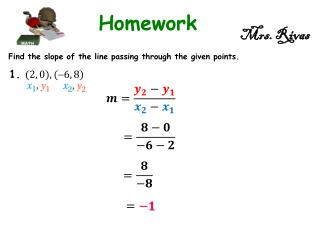DownloadDownload PresentationMrs. Rivas

# Mrs. Rivas

Download Presentation## Mrs. Rivas

- - - - - - - - - - - - - - - - - - - - - - - - - - - E N D - - - - - - - - - - - - - - - - - - - - - - - - - - -
##### Presentation Transcript

1. Mrs. Rivas Find the slope of the line passing through the given points. 1.

2. Mrs. Rivas Find the slope of the line passing through the given points. 2.

3. Mrs. Rivas Find the slope of the line passing through the given points. 3.

4. Mrs. Rivas Find the slope of the line passing through the given points. 4.

5. Mrs. Rivas Find the slope of the line passing through the given points. 5.

6. Mrs. Rivas Graph each line. 6. Starting point

7. Mrs. Rivas Graph each line. 7. Starting point

8. Mrs. Rivas Graph each line. 8. Starting point

9. Mrs. Rivas Graph each line. 9. Starting point

10. Mrs. Rivas Use the given information to write an equation of each line. 10. slope-intercept

11. Mrs. Rivas Use the given information to write an equation of each line. 11. slope-intercept

12. Mrs. Rivas Use the given information to write an equation of each line. 12. slope 5, passes through

13. Mrs. Rivas Use the given information to write an equation of each line. 13. Slope , passes through

14. Mrs. Rivas Use the given information to write an equation of each line. 14. passes through and

15. Mrs. Rivas Use the given information to write an equation of each line. 15. passes through and

16. Mrs. Rivas Write the equations of the horizontal and vertical lines through the given point. 16.

17. Mrs. Rivas Write the equations of the horizontal and vertical lines through the given point. 17.

18. Mrs. Rivas Write the equations of the horizontal and vertical lines through the given point. 18.

19. Mrs. Rivas Write the equations of the horizontal and vertical lines through the given point. 19.

20. Mrs. Rivas Write each equation in slope-intercept form. 20.

21. Mrs. Rivas Write each equation in slope-intercept form. 21.

22. Mrs. Rivas Write each equation in slope-intercept form. 22.

23. Mrs. Rivas Write each equation in slope-intercept form. 23.

24. Mrs. Rivas 24.Coordinate Geometry The vertices of a quadrilateral are , , , and . a. Write an equation for the line through A and B.

25. Mrs. Rivas 24.Coordinate Geometry The vertices of a quadrilateral are , , , and . b. Write an equation for the line through C and D.

26. Mrs. Rivas 24.Coordinate Geometry The vertices of a quadrilateral are , , , and . c. Without graphing the lines, what can you tell about the lines from their slopes? One line has a positive slope and the other has a negative slope. We can also say that they are perpendicular since their slopes are opposite reciprocal.

27. Mrs. Rivas For Exercises 25 and 26, are lines and parallel? Explain. 25. Yes, the lines are parallel because the have the same slopes.

28. Mrs. Rivas For Exercises 25 and 26, are lines and parallel? Explain. 26. No, the lines are NOT parallel because the don’t have the same slopes.

29. Mrs. Rivas Write an equation of the line parallel to the given line that contains. “Same Slope” 27. Use the distributive property Solve for y:

30. Mrs. Rivas Write an equation of the line parallel to the given line that contains. “Same Slope” 28. Use the distributive property Solve for y:

31. Mrs. Rivas Write an equation of the line parallel to the given line that contains. “Same Slope” 29. Use the distributive property Solve for y:

32. Mrs. Rivas Rewrite each equation in slope-intercept form, if necessary. Then determine whether the lines are parallel. Explain. 30. Yes, the lines are parallel because the have the same slopes.

33. Mrs. Rivas Rewrite each equation in slope-intercept form, if necessary. Then determine whether the lines are parallel. Explain. 31. No, the lines are NOT parallel because the don’t have the same slopes.

34. Mrs. Rivas Rewrite each equation in slope-intercept form, if necessary. Then determine whether the lines are parallel. Explain. 32. Yes, the lines are parallel because the have the same slopes.

35. Mrs. Rivas Use slopes to determine whether the opposite sides of quadrilateral WXYZ are parallel. 33. No, the lines are NOT parallel because the don’t have the same slopes.

36. Mrs. Rivas Use slopes to determine whether the opposite sides of quadrilateral WXYZ are parallel. 34. Yes, the lines are parallel because the have the same slopes.

37. Mrs. Rivas For Exercises 35 and 36, are lines and perpendiular? Explain. 35. No, the lines are NOT Perpendicular because the don’t have opposite reciprocal slopes.

38. Mrs. Rivas For Exercises 35 and 36, are lines and perpendiular? Explain. 36. Yes, the lines are Perpendicular because the have opposite reciprocal slopes.

39. Mrs. Rivas Write an equation of the line perpendicular to the given line that contains D. “Opposite Reciprocal slope” 37. Use the distributive property Solve for y:

40. Mrs. Rivas Write an equation of the line perpendicular to the given line that contains D. 38. “Opposite Reciprocal slope” Use the distributive property Solve for y:

41. Mrs. Rivas Write an equation of the line perpendicular to the given line that contains D. 39. “Opposite Reciprocal slope” Use the distributive property Solve for y:

42. Mrs. Rivas Write an equation of the line perpendicular to the given line that contains D. 40. “Opposite Reciprocal slope” Use the distributive property Solve for y: